Select Page

# MCQ in English Answers for CBSE Maths 12 Science Continuity and Differentiability

MCQ in English Answers for CBSE Maths 12 Science Continuity and Differentiability to enable students to get Answers in a narrative video format for the specific question.

Expert Teacher provides MCQ Answers for CBSE Maths 12 Science Continuity and Differentiability through Video Answers in English language. This video solution will be useful for students to understand how to write an answer in exam in order to score more marks. This teacher uses a narrative style for a question from Continuity and Differentiability not only to explain the proper method of answering question, but deriving right answer too.

Please find the question below and view the Answer in a narrative video format.

Question:

## Similar Questions from CBSE, 12th Science, Maths, Continuity and Differentiability

Question 1 : Differentiate the function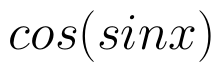with respect to x. (View Answer Video)

Question 2 : Differentiate the function w.r.t.x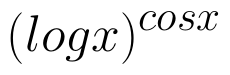. (View Answer Video)

Question 3 : Differentiate w.r.t.x the function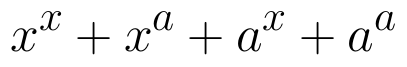, for some fixed a > 0 and x > 0. (View Answer Video)

Question 4 : If x and y are connected parametrically by the equation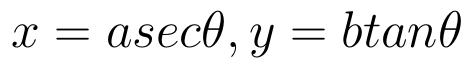, without eliminating the parameter, find. (View Answer Video)

Question 5 : Findfor the function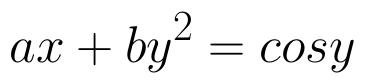. (View Answer Video)

### Integrals

Question 1 : Let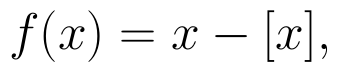for every real number, x, where [x] is the greatest integer less than or equal to x.
Evaluate :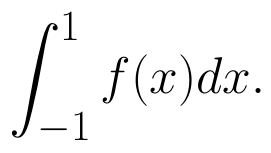(View Answer Video)

Question 2 : Find :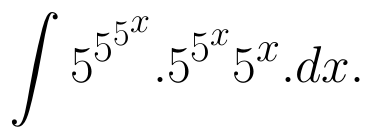(View Answer Video)

Question 3 : Find: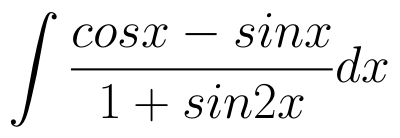. (View Answer Video)

Question 4 : Evaluate: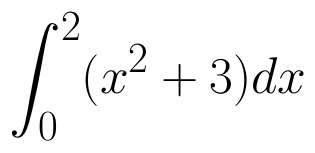as limit of sums.  (View Answer Video)

Question 5 : Find :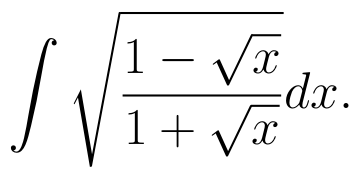(View Answer Video)

### Three Dimensional Geometry

Question 1 : If lines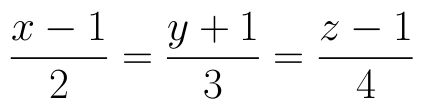and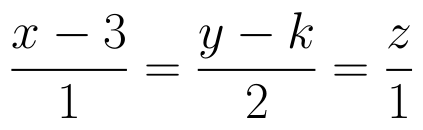intersect, then find the value of k and hence find the equation of plane containing these lines. (View Answer Video)

Question 2 : If a line has the direction ratios -18, 12, -4, then what are its direction cosines? (View Answer Video)

Question 3 : Write the vector equation of a line passing through the point (1, -1, 2) and parallel to the line whose equations are,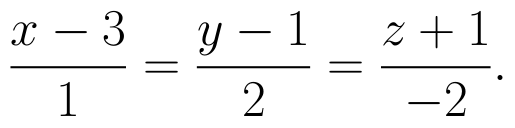(View Answer Video)

Question 4 : Find the distance of the point (2, 12, 5) from the point of intersection of the lines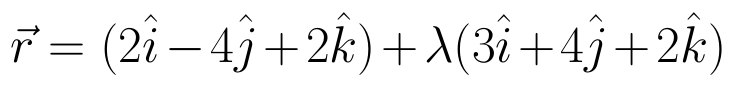and the plane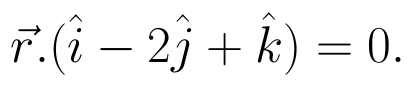(View Answer Video)

Question 5 : Find the distance of the plane : 3x - 4y + 12z = 3 from the origin. (View Answer Video)

### Vector Algebra

Question 1 : Find the value of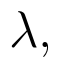if the points with position vectors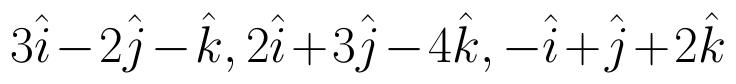and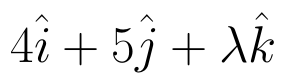are coplanar.  (View Answer Video)

Question 2 : if a, b, c are unit vectors such that a + b + c = 0, then find the value of a.b + b.c + c.a. (View Answer Video)

Question 3 : Find the unit vector in the direction of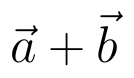if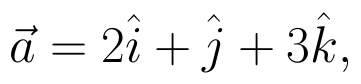and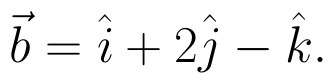(View Answer Video)

Question 4 : Find the angle between the vectors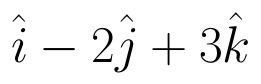and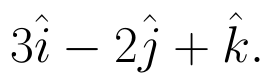(View Answer Video)

Question 5 :  Find a vector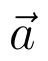of magnitude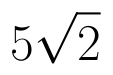making an angle of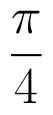with x-axis,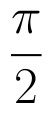with y-axis and an acute angle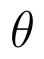with z-axis.  (View Answer Video)